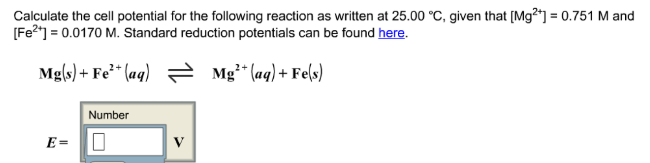# Problem: Calculate the cell potential for the following reaction as written at 25.00°C, given that [Mg2+] = 0.751 M and [Fe2+] = 0.0170 M. Standard reduction potentials can be found here. Mg(s) + Fe2+(aq) ⇌ Mg2+(aq) + Fe(s) E =

###### FREE Expert Solution
80% (492 ratings)###### Problem Details

Calculate the cell potential for the following reaction as written at 25.00°C, given that [Mg2+] = 0.751 M and [Fe2+] = 0.0170 M. Standard reduction potentials can be found here.

Mg(s) + Fe2+(aq) ⇌ Mg2+(aq) + Fe(s)

E =